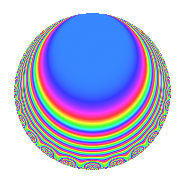# Properties

 Label 4032.2.b.qLevel 4032 Weight 2 Character orbit 4032.b Analytic conductor 32.196 Analytic rank 0 Dimension 8 CM No Inner twists 2

# Related objects

## Newspace parameters

 Level: $$N$$ = $$4032 = 2^{6} \cdot 3^{2} \cdot 7$$ Weight: $$k$$ = $$2$$ Character orbit: $$[\chi]$$ = 4032.b (of order $$2$$ and degree $$1$$)

## Newform invariants

 Self dual: No Analytic conductor: $$32.195682095$$ Analytic rank: $$0$$ Dimension: $$8$$ Coefficient field: 8.0.836829184.2 Coefficient ring: $$\Z[a_1, \ldots, a_{7}]$$ Coefficient ring index: $$2^{9}$$ Sato-Tate group: $\mathrm{SU}(2)[C_{2}]$

## $q$-expansion

Coefficients of the $$q$$-expansion are expressed in terms of a basis $$1,\beta_1,\ldots,\beta_{7}$$ for the coefficient ring described below. We also show the integral $$q$$-expansion of the trace form.

 $$f(q)$$ $$=$$ $$q$$ $$-\beta_{1} q^{5}$$ $$+ \beta_{2} q^{7}$$ $$+O(q^{10})$$ $$q$$ $$-\beta_{1} q^{5}$$ $$+ \beta_{2} q^{7}$$ $$+ ( -\beta_{2} - \beta_{4} - \beta_{7} ) q^{11}$$ $$-\beta_{5} q^{13}$$ $$+ \beta_{1} q^{17}$$ $$+ ( -2 + \beta_{2} - \beta_{4} + \beta_{6} ) q^{19}$$ $$+ ( \beta_{1} - \beta_{2} - \beta_{4} - \beta_{5} ) q^{23}$$ $$+ ( -2 + \beta_{3} + \beta_{6} ) q^{25}$$ $$+ ( -1 + \beta_{2} + \beta_{3} - \beta_{4} + \beta_{6} ) q^{29}$$ $$+ ( -2 - \beta_{6} ) q^{31}$$ $$+ ( -1 - \beta_{1} + \beta_{3} - \beta_{5} ) q^{35}$$ $$+ ( -1 + \beta_{3} - \beta_{6} ) q^{37}$$ $$+ ( \beta_{1} - 2 \beta_{5} ) q^{41}$$ $$+ ( -\beta_{1} + \beta_{2} + \beta_{4} + 2 \beta_{5} + \beta_{7} ) q^{43}$$ $$+ ( -1 - \beta_{2} + \beta_{3} + \beta_{4} ) q^{47}$$ $$+ ( -1 - \beta_{1} + \beta_{2} - \beta_{4} - \beta_{5} + \beta_{6} + \beta_{7} ) q^{49}$$ $$+ ( 4 - 2 \beta_{2} + 2 \beta_{4} - \beta_{6} ) q^{53}$$ $$+ ( -\beta_{2} - 2 \beta_{3} + \beta_{4} - \beta_{6} ) q^{55}$$ $$+ ( 3 + \beta_{2} + \beta_{3} - \beta_{4} ) q^{59}$$ $$+ ( -\beta_{5} - 2 \beta_{7} ) q^{61}$$ $$+ ( -1 - \beta_{2} + \beta_{3} + \beta_{4} ) q^{65}$$ $$+ ( -3 \beta_{1} + \beta_{2} + \beta_{4} + 2 \beta_{5} + \beta_{7} ) q^{67}$$ $$+ ( -\beta_{1} - \beta_{2} - \beta_{4} - \beta_{5} ) q^{71}$$ $$+ ( 2 \beta_{1} - 2 \beta_{5} ) q^{73}$$ $$+ ( 4 + \beta_{1} - 2 \beta_{2} - 2 \beta_{4} - 2 \beta_{5} - \beta_{6} - 2 \beta_{7} ) q^{77}$$ $$+ ( 2 \beta_{1} + \beta_{2} + \beta_{4} + 2 \beta_{5} ) q^{79}$$ $$+ ( 5 - 3 \beta_{2} - \beta_{3} + 3 \beta_{4} ) q^{83}$$ $$+ ( 7 - \beta_{3} - \beta_{6} ) q^{85}$$ $$+ ( -\beta_{1} - 4 \beta_{2} - 4 \beta_{4} - 2 \beta_{5} ) q^{89}$$ $$+ ( -2 - \beta_{1} + \beta_{6} - \beta_{7} ) q^{91}$$ $$+ ( 4 \beta_{1} - 2 \beta_{5} - 2 \beta_{7} ) q^{95}$$ $$+ ( 2 \beta_{5} + 2 \beta_{7} ) q^{97}$$ $$+O(q^{100})$$ $$\operatorname{Tr}(f)(q)$$ $$=$$ $$8q$$ $$\mathstrut +\mathstrut 4q^{7}$$ $$\mathstrut +\mathstrut O(q^{10})$$ $$8q$$ $$\mathstrut +\mathstrut 4q^{7}$$ $$\mathstrut -\mathstrut 8q^{19}$$ $$\mathstrut -\mathstrut 16q^{25}$$ $$\mathstrut -\mathstrut 16q^{31}$$ $$\mathstrut -\mathstrut 8q^{35}$$ $$\mathstrut -\mathstrut 8q^{37}$$ $$\mathstrut -\mathstrut 16q^{47}$$ $$\mathstrut +\mathstrut 16q^{53}$$ $$\mathstrut -\mathstrut 8q^{55}$$ $$\mathstrut +\mathstrut 32q^{59}$$ $$\mathstrut -\mathstrut 16q^{65}$$ $$\mathstrut +\mathstrut 32q^{77}$$ $$\mathstrut +\mathstrut 16q^{83}$$ $$\mathstrut +\mathstrut 56q^{85}$$ $$\mathstrut -\mathstrut 16q^{91}$$ $$\mathstrut +\mathstrut O(q^{100})$$

Basis of coefficient ring in terms of a root $$\nu$$ of $$x^{8}\mathstrut +\mathstrut$$ $$14$$ $$x^{6}\mathstrut +\mathstrut$$ $$61$$ $$x^{4}\mathstrut +\mathstrut$$ $$84$$ $$x^{2}\mathstrut +\mathstrut$$ $$4$$:

 $$\beta_{0}$$ $$=$$ $$1$$ $$\beta_{1}$$ $$=$$ $$($$$$\nu^{5} + 9 \nu^{3} + 16 \nu$$$$)/2$$ $$\beta_{2}$$ $$=$$ $$($$$$\nu^{6} + 11 \nu^{4} + 30 \nu^{2} + 4 \nu + 12$$$$)/4$$ $$\beta_{3}$$ $$=$$ $$($$$$-\nu^{6} - 9 \nu^{4} - 16 \nu^{2} + 2$$$$)/2$$ $$\beta_{4}$$ $$=$$ $$($$$$-\nu^{6} - 11 \nu^{4} - 30 \nu^{2} + 4 \nu - 12$$$$)/4$$ $$\beta_{5}$$ $$=$$ $$($$$$\nu^{5} + 11 \nu^{3} + 26 \nu$$$$)/2$$ $$\beta_{6}$$ $$=$$ $$($$$$-\nu^{6} - 11 \nu^{4} - 26 \nu^{2} + 4$$$$)/2$$ $$\beta_{7}$$ $$=$$ $$($$$$\nu^{7} + 13 \nu^{5} + 50 \nu^{3} + 54 \nu$$$$)/2$$
 $$1$$ $$=$$ $$\beta_0$$ $$\nu$$ $$=$$ $$($$$$\beta_{4}\mathstrut +\mathstrut$$ $$\beta_{2}$$$$)/2$$ $$\nu^{2}$$ $$=$$ $$($$$$\beta_{6}\mathstrut -\mathstrut$$ $$\beta_{4}\mathstrut +\mathstrut$$ $$\beta_{2}\mathstrut -\mathstrut$$ $$8$$$$)/2$$ $$\nu^{3}$$ $$=$$ $$($$$$2$$ $$\beta_{5}\mathstrut -\mathstrut$$ $$5$$ $$\beta_{4}\mathstrut -\mathstrut$$ $$5$$ $$\beta_{2}\mathstrut -\mathstrut$$ $$2$$ $$\beta_{1}$$$$)/2$$ $$\nu^{4}$$ $$=$$ $$($$$$-$$$$7$$ $$\beta_{6}\mathstrut +\mathstrut$$ $$5$$ $$\beta_{4}\mathstrut +\mathstrut$$ $$2$$ $$\beta_{3}\mathstrut -\mathstrut$$ $$5$$ $$\beta_{2}\mathstrut +\mathstrut$$ $$42$$$$)/2$$ $$\nu^{5}$$ $$=$$ $$($$$$-$$$$18$$ $$\beta_{5}\mathstrut +\mathstrut$$ $$29$$ $$\beta_{4}\mathstrut +\mathstrut$$ $$29$$ $$\beta_{2}\mathstrut +\mathstrut$$ $$22$$ $$\beta_{1}$$$$)/2$$ $$\nu^{6}$$ $$=$$ $$($$$$47$$ $$\beta_{6}\mathstrut -\mathstrut$$ $$29$$ $$\beta_{4}\mathstrut -\mathstrut$$ $$22$$ $$\beta_{3}\mathstrut +\mathstrut$$ $$29$$ $$\beta_{2}\mathstrut -\mathstrut$$ $$246$$$$)/2$$ $$\nu^{7}$$ $$=$$ $$($$$$4$$ $$\beta_{7}\mathstrut +\mathstrut$$ $$134$$ $$\beta_{5}\mathstrut -\mathstrut$$ $$181$$ $$\beta_{4}\mathstrut -\mathstrut$$ $$181$$ $$\beta_{2}\mathstrut -\mathstrut$$ $$186$$ $$\beta_{1}$$$$)/2$$

## Character Values

We give the values of $$\chi$$ on generators for $$\left(\mathbb{Z}/4032\mathbb{Z}\right)^\times$$.

 $$n$$ $$127$$ $$577$$ $$1793$$ $$3781$$ $$\chi(n)$$ $$-1$$ $$-1$$ $$1$$ $$1$$

## Embeddings

For each embedding $$\iota_m$$ of the coefficient field, the values $$\iota_m(a_n)$$ are shown below.

For more information on an embedded modular form you can click on its label.

Label $$\iota_m(\nu)$$ $$a_{2}$$ $$a_{3}$$ $$a_{4}$$ $$a_{5}$$ $$a_{6}$$ $$a_{7}$$ $$a_{8}$$ $$a_{9}$$ $$a_{10}$$
3583.1
 − 2.06644i 2.63640i 0.222191i − 1.65222i 1.65222i − 0.222191i − 2.63640i 2.06644i
0 0 0 4.33660i 0 1.65222 2.06644i 0 0 0
3583.2 0 0 0 2.31423i 0 −0.222191 + 2.63640i 0 0 0
3583.3 0 0 0 1.72844i 0 2.63640 + 0.222191i 0 0 0
3583.4 0 0 0 0.922382i 0 −2.06644 1.65222i 0 0 0
3583.5 0 0 0 0.922382i 0 −2.06644 + 1.65222i 0 0 0
3583.6 0 0 0 1.72844i 0 2.63640 0.222191i 0 0 0
3583.7 0 0 0 2.31423i 0 −0.222191 2.63640i 0 0 0
3583.8 0 0 0 4.33660i 0 1.65222 + 2.06644i 0 0 0
 $$n$$: e.g. 2-40 or 990-1000 Embeddings: e.g. 1-3 or 3583.8 Significant digits: Format: Complex embeddings Normalized embeddings Satake parameters Satake angles

## Inner twists

Char. orbit Parity Mult. Self Twist Proved
1.a Even 1 trivial yes
28.d Even 1 yes

## Hecke kernels

This newform can be constructed as the intersection of the kernels of the following linear operators acting on $$S_{2}^{\mathrm{new}}(4032, [\chi])$$:

 $$T_{5}^{8}$$ $$\mathstrut +\mathstrut 28 T_{5}^{6}$$ $$\mathstrut +\mathstrut 196 T_{5}^{4}$$ $$\mathstrut +\mathstrut 448 T_{5}^{2}$$ $$\mathstrut +\mathstrut 256$$ $$T_{11}^{8}$$ $$\mathstrut +\mathstrut 68 T_{11}^{6}$$ $$\mathstrut +\mathstrut 1396 T_{11}^{4}$$ $$\mathstrut +\mathstrut 10272 T_{11}^{2}$$ $$\mathstrut +\mathstrut 18496$$ $$T_{19}^{4}$$ $$\mathstrut +\mathstrut 4 T_{19}^{3}$$ $$\mathstrut -\mathstrut 44 T_{19}^{2}$$ $$\mathstrut -\mathstrut 96 T_{19}$$ $$\mathstrut +\mathstrut 64$$